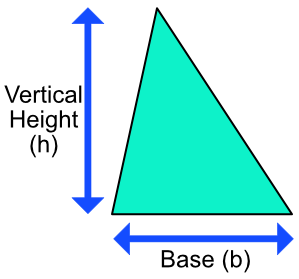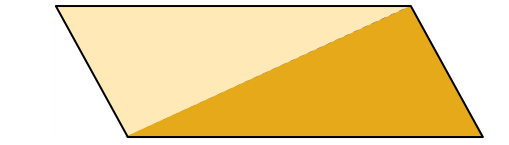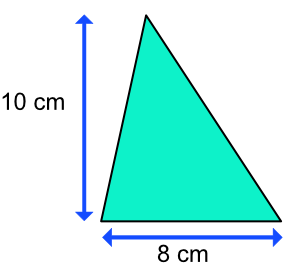Area of a Triangle

## Area of a Triangle

The area of a triangle is given by: frac(1)(2)bhwhere b is the distance along the base of the triangle, and h is the vertical height of the triangle.

You do not need to know this, but this is why it works:

Copy the triangle, and rotate and place it to form a parallelogram.

The area of a parallelogram = base x vertical height.

The area of a triangle is half of that.## Example 1

What is the area of this triangle?Area of a triangle = frac(1)(2)bh = frac(1)(2) xx 10 xx 8 = 40 cm^2

 Area of Triangle = base x height Let the base be x: h = 2x Substitute: 32 = x xx 2x 32 = 2x^2 Divide both sides by 2: 16 = x^2 Square root both sides: 4 = x